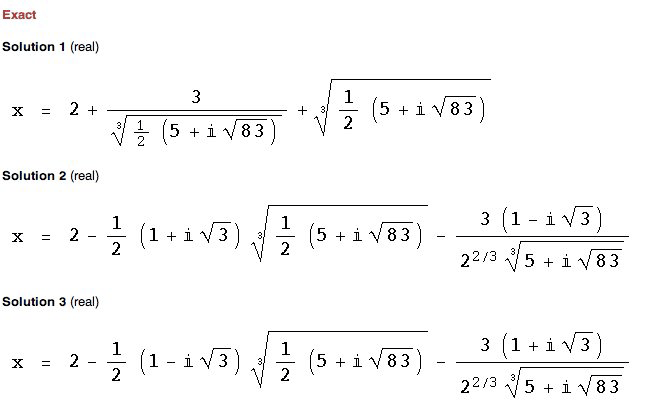SEARCH HOMEMath Central Quandaries & QueriesQuestion from faiz, a student: x3-6x2+3x+5=0Faiz,

Are you sure you have this question stated correctly? There is a procedure for solving cubics similar to the general quadratic expression for quadratics. It's called Cardano's method. This method or something equivalent is what Mathematica uses to solve cubics. When we tried Mathematica with your equation x3 - 6x2 + 3x + 5 = 0 using the Quick Math site we got the followingJanice and HarleyMath Central is supported by the University of Regina and The Pacific Institute for the Mathematical Sciences.